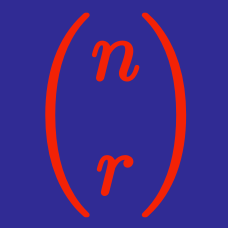Algebra

# Binomial Theorem - Expansions

What is the sum of the coefficients of the terms in $(x-3)^4$ when expanded?

For what value of $n \geq 24$ are the coefficients of $x^{14}$ and $x^{24}$ equal in the expansion of $(1+x)^{n}$?

Expand $(x+1)(x^2-y)^{18}$ and determine the absolute value of the coefficient of $x^{31}y^3$.

What is the coefficient of the $x^3$ term in the expansion of $\left((x+1)^ 2\right)^ {5}$?

The coefficient of $x^{9}y^3$ in the expansion of $(x+ay)^{12}$ is $27500$. What is the value of $a$?

×